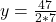## -10\left(-\frac{1}{2}+\left(-\frac{7}{2}\right)+7y=25

Question

-10\left(-\frac{1}{2}+\left(-\frac{7}{2}\right)+7y=25

in progress 0
6 months 2021-08-18T12:06:29+00:00 1 Answers 2 views 0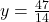Step-by-step explanation:

Given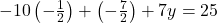Required

Solve for yOpen brackets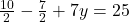Collect Like Terms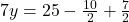Take L.C.M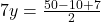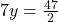Divide both sides by 7